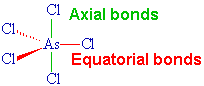# Question #c9022

Feb 23, 2017

Here's what I get for 1. and 2.

#### Explanation:

${\text{PCl}}_{3}$

The Lewis structure of ${\text{PCl}}_{3}$ is(From saylordotorg.github.io)

It has 3 bond pairs and one lone pair for a steric number (SN) of 4.

Hence its electron geometry is tetrahedral.(From OpenStudy)

The molecular shape ignores the lone pair and refers only to the bonds.

Thus, the VSEPR shape of ${\text{PCl}}_{3}$ is trigonal pyramidal.${\text{AsCl}}_{5}$

The Lewis structure of ${\text{AsCl}}_{53}$ isIt has 5 bonding pairs and no lone pairs, so its electron geometry and molecular geometry are the same.The VSEPR shape is trigonal bipyramidal.